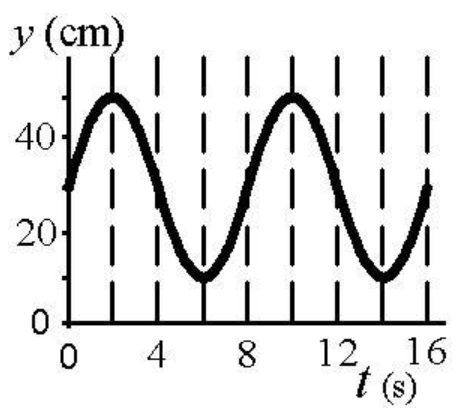# Problem: a. What is the equilibrium position (in cm)?b. What is the maximum velocity of the object (in cm/s)?

###### FREE Expert Solution

a.

The equilibrium position is given by:

$\overline{){{\mathbf{y}}}_{{\mathbf{eq}}}{\mathbf{=}}\frac{{\mathbf{y}}_{\mathbf{max}}\mathbf{+}{\mathbf{y}}_{\mathbf{min}}}{\mathbf{2}}}$

From the diagram, ymax = 50 and ymin = 10

99% (61 ratings)###### Problem Detailsa. What is the equilibrium position (in cm)?
b. What is the maximum velocity of the object (in cm/s)?